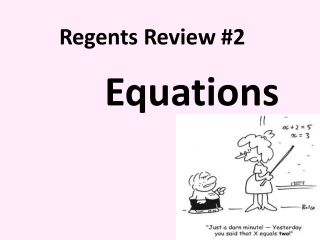DownloadDownload PresentationRegents Review #2

# Regents Review #2

Download Presentation## Regents Review #2

- - - - - - - - - - - - - - - - - - - - - - - - - - - E N D - - - - - - - - - - - - - - - - - - - - - - - - - - -
##### Presentation Transcript

1. Regents Review #2 Equations

2. What type of Equations do we need to solve? Simple Equations Equations with Fractions Quadratic Equations Literal Equations (solving for another variable) Equations that help us solve word problems

3. Simple Equations ½ (2x – 10) = 5x – (6x + 9) x – 5 = 5x – 6x – 9 x – 5 = -x – 9 2x = -4 x = -2 Always check solution(s) to any equation ½ (2 -2 – 10) = 5(-2) – (6 -2 + 9) ½ (-14) = -10 – (-3) -7 = -7 It checks!

4. How do we solve Quadratic Equations? 1) x2 = a Example: x2 = 16 Take the square root of both sides x = x = 4 or x = {4,-4} • x2 + bx + c = 0 Example: x2 – 5x + 6 = 0 Set all terms equal to zero (x – 2)(x – 3)= 0 Factor x – 2 = 0 x – 3 = 0 Set each factor equal to zero x = 2 x = 3 Solve x = {2,3}

5. Equations with Fractions There are two ways to “clear” fractions in Equations… • Multiply both sides of the equation by the LCD (Least Common Denominator) 2) Cross Multiply (only works for proportions )

6. Equations with Fractions Cross Multiplication (solving proportions) Check for solutions that will make the denominator equal to zero. x = {3,1}

7. Equations With Fractions Multiplying by the LCD CHECK

8. Literal Equations When solving literal equations, isolate the indicated variable using inverse operations

9. Word Problems We can use equations to solve many different types of word problems. When solving a word problem, remember to… • Define all unknowns (set up “Let” statements) • Write an equation relating all unknowns • Solve the equation • Determine the value of all unknowns • Answer the question (use appropriate units)

10. Word Problems Find two consecutive integers whose sum is -35. -18 -17 (-18 + 1) x: 1st consecutive integer x + 1: 2nd consecutive integer Remember: Consecutive integers count by 1’s Ex: x, x+1, x+2, x+3…. Consecutive odd or even integers count by 2’s Ex: x, x+2, x+4, x+6…

11. Word Problems Ticket sales for a music concert totaled \$2,160. Three times as many tickets were sold for the Saturday night concert than the Sunday afternoon concert. Two times as many tickets were sold for the Friday night concert than the Sunday afternoon concert. Tickets for all three concerts sold for \$2 each. Find the number of tickets sold for the Saturday night concert. x: number of tickets sold for the Sunday concert 3x: number of tickets sold for the Saturday concert 2x: number of tickets sold for the Friday concert 180 tickets 540 tickets 3(180) 360 tickets 2(180) 2x + 2(3x) + 2(2x) = 2160 There were 540 tickets sold for the Saturday night concert 2x + 6x + 4x = 2160 12x = 2160 x = 180

12. Word Problems A person has 23 coins made up of dimes and quarters worth \$3.35. How many coins of each type are there? 0.10x + 0.25(23 – x) = 3.35 16 dimes 7 quarters (23 – 16) 10x + 25(23 – x) = 335 10x + 575 – 25x = 335 -15x + 575 = 335 -15x = -240 x = 16 Check 7(25) + 16(10) = 335 175 + 160 = 335 335 = 335

13. Word Problems The area of a rectangle is 120 square inches. If the length is two more than the width, find the dimensions of the figure. A = lw 120 = (x + 2)(x) 120 = x2 + 2x 0 = x2 + 2x – 120 0 = (x + 12)(x – 10) x + 12 = 0 x – 10 = 0 x = -12 x = 10 Reject Width: x Length: x + 2 The width is 10 inches The length is 12 inches

14. Word Problems Distance, Rate, Time (D = RT) • Two Options • Finding Rate • 2) Finding Time D R T How do you know what to look for? Distance: How far? Rate: How fast? Time: How long?

15. Word Problems Hannah took a trip to her cousin’s house. She drove 120 miles to get there. If it took her 1.2 hours to get halfway to her cousin’s house, what was her average rate of speed? Important Information: Distance traveled: 60 miles (120/2) Time: 1.2 hours Rate: ? If Hannah decided to travel 10 mph faster on the way home, how long would it take her?

16. Now it’s your turn to review on your own! Using the information presented today and your review packet, complete the practice problems in the packet.Regents Review #3 is FRIDAY, May 17thBE THERE!!!!